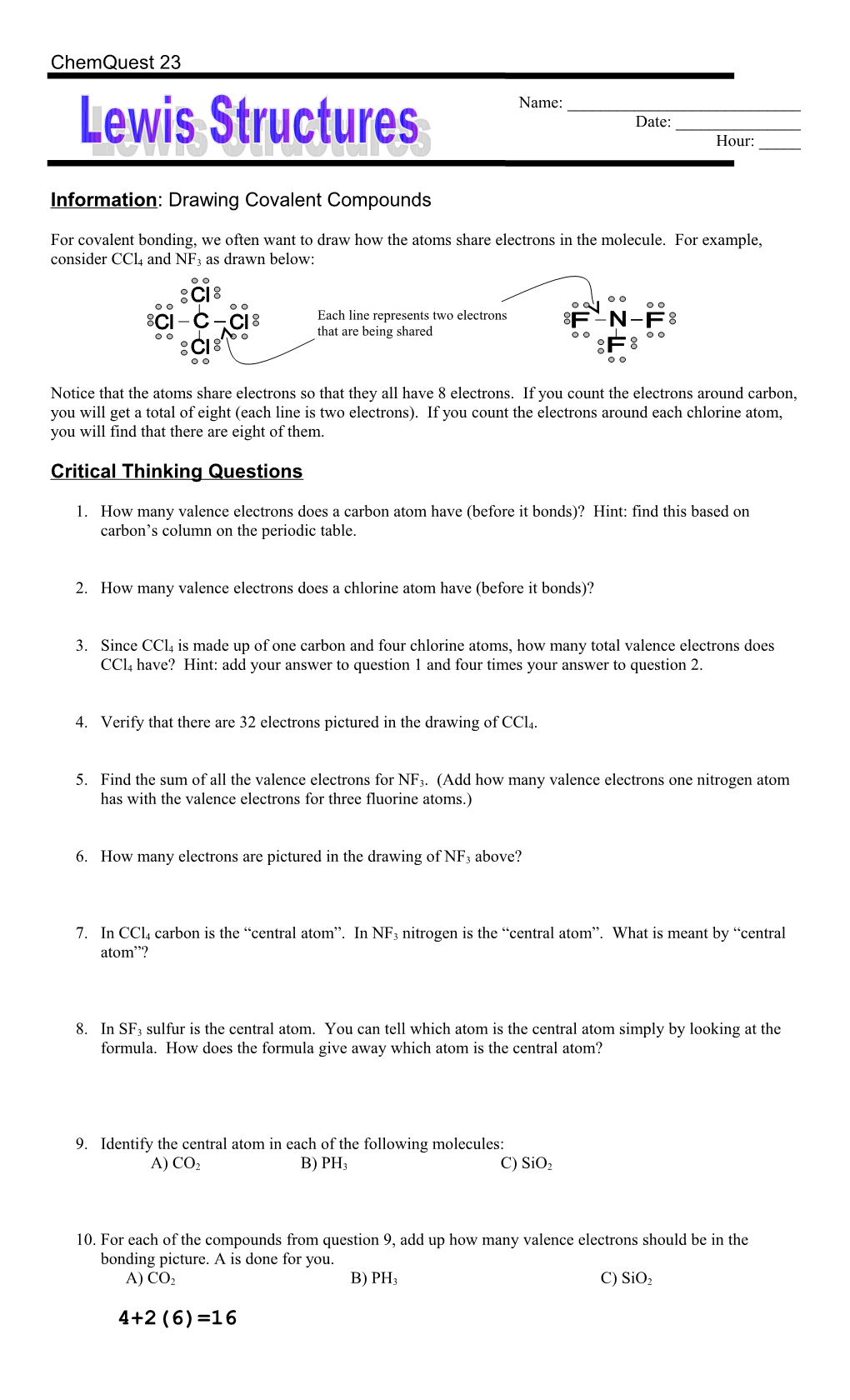# Information: Drawing Covalent CompoundsChemQuest 23

Name: ______

Date: ______

Hour: _____

Information: Drawing Covalent Compounds

For covalent bonding, we often want to draw how the atoms share electrons in the molecule. For example, consider CCl4 and NF3 as drawn below:

Notice that the atoms share electrons so that they all have 8 electrons. If you count the electrons around carbon, you will get a total of eight (each line is two electrons). If you count the electrons around each chlorine atom, you will find that there are eight of them.

Critical Thinking Questions

1. How many valence electrons does a carbon atom have (before it bonds)? Hint: find this based on carbon’s column on the periodic table.
1. How many valence electrons does a chlorine atom have (before it bonds)?
1. Since CCl4 is made up of one carbon and four chlorine atoms, how many total valence electrons does CCl4 have? Hint: add your answer to question 1 and four times your answer to question 2.
1. Verify that there are 32 electrons pictured in the drawing of CCl4.
1. Find the sum of all the valence electrons for NF3. (Add how many valence electrons one nitrogen atom has with the valence electrons for three fluorine atoms.)
1. How many electrons are pictured in the drawing of NF3 above?
1. In CCl4 carbon is the “central atom”. In NF3 nitrogen is the “central atom”. What is meant by “central atom”?
1. In SF3 sulfur is the central atom. You can tell which atom is the central atom simply by looking at the formula. How does the formula give away which atom is the central atom?
1. Identify the central atom in each of the following molecules:

A) CO2B) PH3C) SiO2

1. For each of the compounds from question 9, add up how many valence electrons should be in the bonding picture. A is done for you.

A) CO2B) PH3C) SiO2

4+2(6)=16

1. The number of electrons that should appear in the bonding picture for CO3 is 22. The number of electrons that appear in the picture for CO32- is 24. Offer an explanation for why CO32- has 24 electrons instead of 22. (Where did the extra two electrons come from?)
1. The number of electrons that should be included in the picture of NH4 is 9. The number of electrons in the picture for NH4+ is 8. Offer an explanation for why NH4+ has 8 electrons instead of 9.
1. Considering questions 11 and 12, we can formulate a rule: For each negative charge on a

polyatomic ion, we must ______an electron and for each positive charge we must

______an electron.

1. For each of the polyatomic ions or molecules below, determine the total number of valence electrons.

a) NO3-b) SCl4c) H3O+d) PO43-

Information: Steps for Drawing Lewis Structures for Covalent Compounds

Study the two examples in the table of how to write structures for CO32- and NH3. Make sure you understand each of the five steps.

CO32- / NH3
Step #1: Add up the number of valence electrons that should be included in the Lewis Structure. / 4 + 3(6) + 2 = 24
(carbon has four and each oxygen has six; add two for the -2 charge) / 5 + 3(1) = 8
(nitrogen has five; each hydrogen has one)
Step #2: Draw the “skeleton structure” with the central atoms and the other atoms, each connected with a single bond.
Step #3: Add six more electron dots to each atom except the central atom. Also, never add dots to hydrogen. / (no change)
Step #4: Any “leftover” electrons are placed on the central atom. Find the number of leftovers by taking the total from Step #1 and subtracting the number of electrons pictured in Step #3. / 24 – 24 = 0 leftover electrons
(no change) / 8 – 6 = 2 leftover electrons; placed around nitrogen
Step #5: If the central atom has 8, then you are done. If not, then move two electrons from a different atom to make a multiple bond. Keep making multiple bonds until the central atom has 8 electrons. / / (no change)

Critical Thinking Questions

1. Write the Lewis Structure for nitrate, NO3-1. Hint: when you are done it should look very similar to CO32- in the table above.
1. Draw the Lewis Structure for SO2.